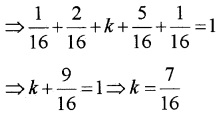# Plus Two Maths Chapter Wise Questions and Answers Chapter 13 Probability

Students can Download Chapter 13 Probability Questions and Answers, Plus Two Maths Chapter Wise Questions and Answers helps you to revise the complete Kerala State Syllabus and score more marks in your examinations.

## Kerala Plus Two Maths Chapter Wise Questions and Answers Chapter 13 Probability

### Plus Two Maths Probability Three Mark Questions and Answers

Question 1.
Determine P(E/F). A die is thrown three times, E: ‘4 appears on the third toss’, F: ‘6 and 5 appears respectively on the two tosses’.
n(S) = 63 = 216
E = {( 1, 1, 4), (1, 2, 4), (1, 3, 4)……….(1, 6, 4),
(2, 1, 4), (2, 2, 4), (2, 3, 4)……..(2, 6, 4),
(3, 1, 4), (3, 2, 4), (3, 3, 4)……..(3, 6, 4),
(4, 1, 4), (4, 2, 4), (4, 3, 4)…….(4, 6, 4),
(5, 1, 4), (5, 2, 4), (5, 3, 4)……..(5, 6, 4),
(6, 1, 4), (6, 2, 4), (6, 3, 4)……..(6, 6, 4)}
F = {(6, 5, 1), (6, 5, 2), (6, 5, 3), (6, 5, 4), (6, 5, 5), (6, 5, 6)}
⇒ E ∩ F = {(6, 5, 4)}
P(F) = $$\frac{6}{216}$$ and P(E ∩ F) = $$\frac{1}{216}$$
Then, P(E/F) = $$\frac{P(E \cap F)}{P(F)}=\frac{\frac{1}{216}}{\frac{6}{216}}=\frac{1}{6}$$.Question 2.
Determine P(E/F). Mother, Father and son lineup at random for a photograph.
E: ‘Son on one end’, F: ‘ Father in middle.
Let Mother-M, Father-F and Son-S.
n(S) = 3! = 6
E = {SMF, SFM, MFS, FMS},
F = {MFS, SFM}
⇒ E ∩ F = {SFM, MFS}
P(F) = $$\frac{2}{6}$$ = $$\frac{1}{3}$$ and P(E ∩ F) = $$\frac{2}{6}$$ = $$\frac{1}{3}$$
Then, P(E/F) = $$\frac{P(E \cap F)}{P(F)}=\frac{\frac{1}{3}}{\frac{1}{3}}=1$$.

Question 3.
A black and a red dice are rolled

1. Find the conditional probability of obtaining a sum greater than 9, given that the black die resulted in a 5.
2. Find the conditional probability of obtaining the sum 8, given that the red die resulted in a number less than 4.

We have, n(S) = 36
1. E = Event of 5 on black die.
E = {(5, 1), (5, 2), (5, 3), (5, 4), (5, 5), (5, 6)}
P(E) = $$\frac{6}{36}$$ = $$\frac{1}{6}$$
F = Getting a sum greater than 9.
F = {(4, 6), (5, 5), (6, 4)(5, 6), (6, 5), (6, 6)}
⇒ E ∩ F = {(5,5), (5,6)}
P(E ∩ F) = $$\frac{2}{36}$$= $$\frac{1}{18}$$
Therefore the required probability
P(E/F) = $$\frac{P(E \cap F)}{P(F)}=\frac{\frac{1}{18}}{\frac{1}{6}}=\frac{1}{3}$$.

2. E = Event of a number less than 4 on red die.
E = {(1, 1), (1, 2), (1, 3), (2, 1), (2, 2), (2, 3),
(3, 1), (3, 2), (3, 3), (3, 1), (3, 2), (3, 3),
(5, 1), (5, 2), (5, 3), (6, 1), (6, 2), (6, 3)}
P(E) = $$\frac{18}{36}$$ = $$\frac{1}{2}$$
F = Getting a sum 8.
F = {(4, 4), (5, 3), (3, 5)(2, 6), (6, 2), (6, 6)}
⇒ E ∩ F = {(5, 3),(6, 2)}
P(E ∩ F) = $$\frac{2}{36}$$= $$\frac{1}{18}$$
Therefore the required probability
P(E/F) = $$\frac{P(E \cap F)}{P(E)}=\frac{\frac{1}{18}}{\frac{1}{2}}=\frac{1}{9}$$.Question 4.
An instructor has a question bank consisting of 300 easy True/False questions, 200 difficult true/False questions, 500 easy multiple choice questions, and 400 difficult multiple choice questions. If a question is selected from the test question bank, what is the probability that it will be an easy question given that it is a multiple choice question?
Describe the events as follows
E: ‘getting an easy question.’
F: ‘getting a multiple choice question.’
Total Questions = 300 + 200 + 500 + 400 = 1400
n(F) = 500 + 400 = 900, n(E ∩ F) = 500
P(F) = $$\frac{900}{1400}=\frac{9}{14}$$, P(E ∩ F) = $$\frac{500}{1400}=\frac{5}{14}$$
Therefore the required probability
= P(E/F) = $$\frac{P(E \cap F)}{P(F)}=\frac{\frac{5}{14}}{\frac{9}{14}}=\frac{5}{9}$$.

Question 5.
Two cards are drawn at random without replacement from a pack of 52 playing cards. Find the probability that both the cards are black.
Describe the events as follows
B1: ‘getting a black card in the first draw.’
B2: ‘getting a black card in the second draw.’
P(B1) = $$\frac{26}{52}$$ = $$\frac{1}{2}$$
When the first event is executed and since no replacement is allowed, the remaining total number of cards become 51 and black cards become 25.
P(B2/B1) = $$\frac{25}{51}$$
Therefore the required probability
P(B1 ∩ B2) = P(Bl) × P(B2/B1) = $$\frac{1}{2} \times \frac{25}{51}=\frac{25}{102}$$.Question 6.
If P(A) = $$\frac{6}{11}$$, P(B) = $$\frac{5}{11}$$ and P(A ∪ B) = $$\frac{9}{11}$$ Find

1. P(A ∩ B)
2. P(A/B)
3. P(B/A)

1. P(A ∩ B) = P(A) + P(B) – P(A ∪ B)2. P(A/B)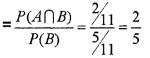3. P(B/A)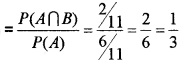Question 7.
Events A and B are such that P(A) = $$\frac{1}{2}$$, P(B) = $$\frac{7}{12}$$ and P(not A or not B) = $$\frac{1}{4}$$ State whether A and B are independent.
P(not A or not B) = $$\frac{1}{4}$$ ⇒ $$P(\bar{A} \cup \bar{B})=\frac{1}{4}$$We have, P(A) × P(B) = $$\frac{1}{2} \times \frac{7}{12}=\frac{7}{24}$$
Therefore, P(A ∩ B) ≠ P(A) × P(B)
Hence A and B are not independent.Question 8.
Consider two events such that P(A) = $$\frac{1}{2}$$, P(A ∪ B) = $$\frac{3}{5}$$ and P(B) = p. Find p, if A and B are independent events.
If A and B are independent then
P(A ∩ B) = P(A) × P(B)
We have,
P(A ∪ B) = P(A) + P(B) – P(A) × P(B)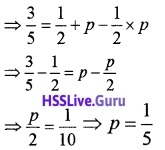Question 9.
One card is drawn at random from a well shuffled pack of 52 cards. In which of the following cases are the events E and F independent? (3 scores each)

1. E: ‘the card drawn is a spades.’
F: ‘the card drawn is an ace.’
2. E: ‘the card drawn is a black.’
F: ‘the card drawn is a king.’
3. E: ‘the card drawn is a king or a queen.’
F: ‘the card drawn is queen or a jack.’

1. P(E) = $$\frac{13}{52}=\frac{1}{4}$$, P(F) = $$\frac{4}{52}=\frac{1}{13}$$
There is only one card which is an ace of spade.
P(E ∩ F) = $$\frac{1}{52}$$
We have,
P(E) × P(F) = $$\frac{1}{4} \times \frac{1}{13}=\frac{1}{52}$$ = P(E ∩ F)
Hence E and F are independent events.

2. P(E) = $$\frac{26}{52}=\frac{1}{2}$$, P(F) = $$\frac{4}{52}=\frac{1}{13}$$
There are two king of black.
P(E ∩ F) = $$\frac{2}{52}$$ = $$\frac{1}{26}$$
We have,
P(E) × P(F) = $$\frac{1}{2} \times \frac{1}{13}=\frac{1}{26}$$ = P(E ∩ F)
Hence E and F are independent events.

3. There are 4 king and 4 queen cards
P(E) = $$\frac{8}{52}$$ = $$\frac{2}{13}$$,
There are 4 queen and 4 jack cards.
P(F) = $$\frac{8}{52}$$ = $$\frac{2}{13}$$
There 4 queen common for both.
P(E ∩ F) = $$\frac{4}{52}$$ = $$\frac{1}{13}$$
We have,
P(E) × P(F) = $$\frac{2}{13} \times \frac{2}{13}=\frac{4}{169}$$ ≠ P(E ∩ F)
Hence E and F are not independent events.Question 10.
A fair coin and an unbiased die are tossed. Let A be the event ‘head appears on the coin’ and B be the event ‘3 on the die’. Check whether A and B are independent events or not.
P(A) = $$\frac{1}{2}$$ and P(B) = $$\frac{1}{6}$$
When a coin and die are tossed the sample space will be as follows.
S = {H1, H2, H3, H4, H5, H6, T1, T2, T3, T4, T5, T6}
Here head and 3 come only once.
⇒ P(A ∩ B) = $$\frac{1}{12}$$
P(A) × P(B) = $$\frac{1}{2} \times \frac{1}{6}=\frac{1}{12}$$ = P(A ∩ B)
Hence A and B are independent.

Question 11.
Rani and Joy appear in an interview for two vacancies in the same post. The probability of Rani’s selection is $$\frac{1}{7}$$ and that of Joy’s selection is $$\frac{1}{5}$$ .What is the probability that

1. Rani will not be selected? (1)
2. Both of them will be selected? (1)
3. None of them will be selected? (1)

1. Let Rani’s selection be the event A and Joy’s selection be the event B.P(Rani will not be selected)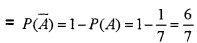2. P(Both of them will be selected)
P(A ∩ B) = P(A).P(B) = $$\frac{1}{7} \cdot \frac{1}{5}=\frac{1}{35}$$.

3. P(None selected)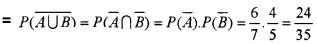Question 12.
Find the probability distribution of number heads in two tosses of a coin.
S = {HH, HT, TH, TT}
Let X denotes the random variable of getting a head. Then X can take values 0, 1, 2.
P(X = 0) = P(no heads) = P({TT})
= P(T) × P(T) = $$\frac{1}{2} \times \frac{1}{2}=\frac{1}{4}$$
P(X = 1) = P(one heads)
= P({HT, TH})
= P(H) × P(T) + P(T) × P(H)
= $$\frac{1}{2} \times \frac{1}{2}+\frac{1}{2} \times \frac{1}{2}=\frac{1}{2}$$
P(X = 2) = P(two heads) = P({HH})
= P(H) × P(H) = $$\frac{1}{2} \times \frac{1}{2}=\frac{1}{4}$$
The required Probability Distribution is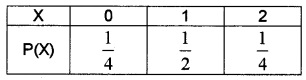Question 13.
Ten eggs are drawn successively with replacement from a lot containing 10% defective eggs. Find the probability that there is at least one defective egg.
Let X denotes the random variable of number of defective eggs in the 10 eggs drawn.
Clearly X has a Binomial Distribution with n = 10 and p = 10% = $$\frac{1}{10}$$, q = 1 – p = $$\frac{9}{10}$$
⇒ P(X = x) = 10Cxq10-xpx = 10Cx$$\left[\frac{9}{10}\right]^{10-x}\left[\frac{1}{10}\right]^{x}$$
P(at least 1 defective egg) = P(X ≥ 1)
1 – P(X = 0) = 1 – 10C0$$\left[\frac{9}{10}\right]^{10}=1-\frac{9^{10}}{10^{10}}$$.

### Plus Two Maths Probability Four Mark Questions and Answers

Question 1.
In a hostel 50 % of the girls like tea, 40 % like coffee and 20% like both tea and coffee. A girl is selected and random.

1. Find the probability that she likes neither tea nor coffee. (2)
2. If the girl likes tea, then find the probability that she likes coffee. (1)
3. If she likes coffee then find the probability she likes tea. (1)

Let T denotes the set of girls who like tea and C denotes who like coffee.
1. P(T) = 50% = $$\frac{1}{2}$$; P(C) = 40% = $$\frac{2}{5}$$;
P(T ∩ C) = 20% = $$\frac{1}{5}$$
P(T ∪ C) = 1 – P(T ∪ C)
= 1 – {P(T) + P(C)-P(T ∩ C)}
$$=1-\left\{\frac{1}{2}+\frac{2}{5}-\frac{1}{5}\right\}=\frac{3}{10}$$.

2.3.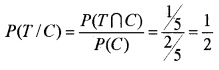Question 2.
A box of oranges is inspected by examining three randomly selected oranges drawn without replacement. If all the three oranges are good, the box is approved for sale, otherwise, it is rejected. Find the probability that a box containing 15 oranges out of which 12 are good and 3 are bad ones will be approved for sale.
For the box to be approved all the three oranges should be selected from the 12 good ones. Since the events are executed without replacement the number for good oranges and total oranges reduce by one on each draw.
O1: ‘getting a good orange in the first draw.’
O2: ‘getting a good orange in the second draw.’
O3: ‘getting a good orange in the third draw.’
P(good orange in the first draw) =P(O1)= $$\frac{12}{15}$$ = $$\frac{4}{5}$$,
P(good orange in the second) = p(O2/O1) = $$\frac{11}{14}$$,
P(good orange in the third)
= P(O3/(O1 ∩ O2)) = $$\frac{10}{13}$$
Therefore the required probability
= P(O1 ∩ O2 ∩ O3)
= P(O1)P(O2/O1)P(O3/(O1 ∩ O2))
$$=\frac{4}{5} \times \frac{11}{14} \times \frac{10}{13}=\frac{44}{91}$$.

Question 3.
Let two independent events A and B such that P(A) = 0.3, P(B) = 0.6. Find

1. P(A and B)
2. P(A and not B)
3. P(A or B)
4. P (neither A nor B)

1. P(A and B) = P(A ∩ B) = P(A) × P(B)
= 0.3 × 0.6 = 0.18.

2. P(A and not B) = P(A ∩ $$\bar{B}$$) = P(A) × P($$\bar{B}$$)
= 0.3 × 0.4 = 0.12.

3. P(A or B) = P(A ∪ B)
= P(A) + P(B) – P(A ∩ B)
= P(A) + P(B) – P(A) × P(B)
= 0.3 + 0.6 – 0.3 × 0.6 = 0.72.

4. P(neither A nor B) = $$P(\bar{A} \cap \bar{B})$$
= P($$\bar{A}$$) × P($$\bar{B}$$) = 0.7 × 0.4 = 0.28.Question 4.
Two balls are drawn at random with replacement from a box containing 10 black and 8 red balls. Find the probability that

1. both balls are red.
2. the first ball is a black and the second is red
3. one of them is black and the other red.

Describe the events as follows.
Black-B and Red-R.n(S) = 18,
P(B) = $$\frac{10}{18}$$ = $$\frac{5}{9}$$ and P(R) = $$\frac{8}{18}$$ = $$\frac{4}{9}$$
Since the event is executed with replacement, is independent.
1. P( both ball is red) = P(R) × P(R)
= $$\frac{4}{9} \times \frac{4}{9}=\frac{16}{81}$$.

2. P( first black and second red) = P(B) × P(R)
= $$\frac{5}{9} \times \frac{4}{9}=\frac{20}{81}$$.

3. P( one of them is a black and the other red)
= P(B) × P(R) + P(R) × P(B)
$$=\frac{5}{9} \times \frac{4}{9}+\frac{4}{9} \times \frac{5}{9}=\frac{40}{81}$$.

Question 5.
Bag 1 contains 3 red and 4 black balls while another Bag II contains 5 red and 6 black balls. One ball is drawn at random from one of the Bags and it is found to be red. Find the probability that it was from Bag II.
Describe the events as follows.
A: ‘getting a defective ball’.
E1: ‘choosing Bag I.’
E2: ‘choosing Bag II.’
P(E1) = P(E2) = $$\frac{1}{2}$$
P(A/E1) = P (drawing a red ball from Bag I) = $$\frac{3}{7}$$
P(A/E2) = P (drawing a red ball from Bag II) = $$\frac{5}{11}$$
P (a ball from Bag II, being given that it is red)Question 6.
A Bag contains 4 red and 4 black balls, another bag contains 2 red and 6 black balls. One ball of the two Bag is drawn at random and the ball is drawn the Bag is found to be red. Find the probability that the ball is drawn from first Bag.
Describe the events as follows.
A: ‘getting a red ball’.
E1: ‘choosing Bag I.’
E2: ‘choosing Bag II.’
P(E1) = P(E2) = $$\frac{1}{2}$$
P(A/E1) = P (a red ball from Bag I) = $$\frac{4}{8}$$ = $$\frac{1}{2}$$
P(A/E2) = P (a red ball from Bag II) = $$\frac{2}{8}$$ = $$\frac{1}{4}$$
P (a ball from Bag II, being given that it is red)Question 7.
In a factory which manufactures blots, machines A, B, and C manufacture respectively 25%, 35%and 40% of the bolts. Of their outputs 5%, 4% and 2%are defective bolts. A bolt is drawn at random from the product and is found to be defective. What is the probability that it is manufactured by the machine B?
Describe the events as follows.
A: ‘getting a defective bolt’.
E1: ‘choosing machine A.’
E2: ‘choosing machine B.’
E3: ‘choosing machine C.’
P(E1) = 0.25, P(E2) = 0.35, P(E3) = 0.4
P(A/E1) = P
(a defective bolt from machine A)
= 5% = 0.05
P(A/E2) = P
(a defective bolt from machine B)
= 4% = 0.04
P(A/E3) = P
(a defective bolt from machine C)
= 2% = 0.02
P (a bolt from machine B, being given that it is defective)
= P(E2/A)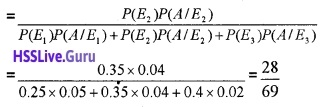Question 8.
Suppose 5% of men and 0.25% of women have hair grey hair. A grey haired person is selected at random. What is the probability of this person being male? Assume there are equal number males and females.
Describe the events as follows.
A: ‘person is grey haired’.
E1: ‘choosing man.’
E2: ‘choosing woman.’
P(E1) = P(E2) = $$\frac{1}{2}$$
P(A/E1) = P (a grey haired person from men)
= 5% = 0.05
P(A/E2) = P (a grey haired person from women) = 0.25% = 0.0025
P(selecting a male, being given that it is grey haired)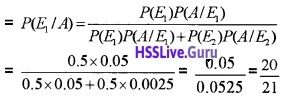Question 9.
An Insurance company insured 2000 scooter drivers, 4000 car drivers, and 6000 truck drivers. The probabilities of an accident are .01, 0.03 and 0.15 respectively. One of the insured persons meets with an accident. What is the probability that he is a scooter driver?
Describe the events as follows.
A: ‘accident happens’.
E1: ‘choosing Scooter driver.’
E2: ‘choosing Car driver.’
E3: ‘choosing Truck driver.’
Total drivers = 2000 + 4000 + 6000 = 12000P(A/E1) = P
(accident of a Scooter driver) = 0.01
P(A/E2) = P
(accident of a Car driver) = 0.03
P(A/E3) = P
(accident of a Truck driver) = 0.15
P (accident happens, given that it is a Scooter driver).
= P(E1/A)Question 10.
A factory has two machines A and B. Past record shows that machine A produced 60% of the items of output and machine B produced 40% of the items. Further, 2% of the items produced by machine A and 1% produced by machine B were defective. All the items are put into one stockpile and then one item is chosen at random from this and is found to be defective. What is the probability that it was produced by machine B?
Describe the events as follows.
A: ‘getting a defective item.’
E1: ‘choosing machine A.’
E2: ‘choosing machine B.’
P(E1) = 60% = 0.6, P(E2) = 40% = 0.4
P(A/E1) = P (a defective from machine A) = 2% = 0.02
P(A/E2) = P (a defective from machine B) = 1% = 0.01
P (a defective from machine B)Question 11.
Suppose a girl throws a die. If she gets a 5 or 6, she tosses a coin three times and notes the number of heads. If she gets 1, 2, 3 or 4 she tosses a coin once and notes whether a head or tail is obtained. If she obtained exactly one head, what is the probability that she threw 1, 2, 3 or 4 with the die?
Describe the events as follows.
E1: ‘she getting 5 or 6.’
E2: ‘she getting 1, 2, 3 or 4.’
When a die is thrown the sample space is{1, 2, 3, 4, 5, 6}
P(E1) = $$\frac{2}{6}$$ = $$\frac{1}{3}$$, P(E2) = $$\frac{4}{6}$$ = $$\frac{2}{3}$$
When she gets 5 or 6, throws a coin 3 times. Then sample space is {HHH, HHT, HTH, THH, TTH, THT, HTT, TTT}
P(A/E1) = P (one head given that 5 or 6 happened)
$$\frac{3}{8}$$
When she gets 1, 2, 3 or 4, throws a coin once. Then sample space is {H, T}
P(A/E2) = P
(one head given that 1, 2, 3 or 4 happened)
$$\frac{1}{2}$$
P (She gets exactly one head threw 1, 2, 3 or 4)Question 12.
Vineetha and Reshma are competing for the post of school leader. The probability Vineetha to be elected is 0.6 and that of Reshma is 0.4 Further if Vineetha is elected the probability of introducing a new pattern of election is 0.7 and the corresponding probability is 0.3 if Resma is elected. Find the probability that the new pattern of election is introduced by Reshma.
Let E1 and E2 be the respectively probability that Vineetha and Reshma will be elected. Let Abe the probability that a new pattern of election is introduced.
P(E1) = 0.6; P(E2) = 0.4
P(A|E1) = 0.7; P(A|E2) = 0.3Question 13.
Find the probability of number of doublets in three throws of a pair of dice.
Let X denotes the random variable of getting a Doublet. Possible doublets are (1, 1),(2, 2),(3, 3),(4, 4),(5, 5),(6, 6).
Then X can take values 0, 1, 2, 3.
P(getting a doublet) = $$\frac{6}{36}$$ = $$\frac{1}{6}$$
P(not getting a doublet) = $$\frac{30}{36}$$ = $$\frac{5}{6}$$
P(X = 0) = P(no doublet) = $$\frac{5}{6} \times \frac{5}{6} \times \frac{5}{6}=\frac{125}{216}$$
P(X = 1) = P(one doublet and 2 non-doublets)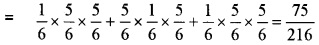P(X = 2) = P(2 doublet and 1 non-doublets)P(X = 3) = P(3 doublet) = $$\frac{1}{6} \times \frac{1}{6} \times \frac{1}{6}=\frac{1}{216}$$
The required Probability Distribution is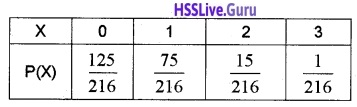Question 14.
Find the probability distribution of the number of white balls drawn when three balls are drawn one by one without replacement from a bag containing 4 white and 6 red balls.
Let X denotes the random variable of number of white balls. Clearly X can take values 0, 1, 2, 3. Describe the events as follows.
W: ‘getting white ball.’
R: ‘getting red ball.’
P(X=0) = P(no white balls)
= P(RRR)= $$\frac{6}{10} \times \frac{5}{9} \times \frac{4}{8}=\frac{5}{30}$$
P(X=1) = P(1white, 2red balls)
= P(WRR) + P(RWR) + P(RRW)P(X=2) = P(2white, 1 red balls)
= P(WWR) + P(RWW) + P(WRW)P(X=3) = P(3white)
P(WWW) = $$\frac{4}{10} \times \frac{3}{9} \times \frac{2}{8}=\frac{1}{30}$$
The required Probability Distribution is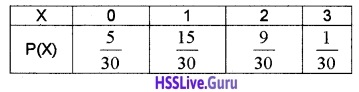Question 15.
Two dice are thrown simultaneously. If X denotes the number of sixes, find expectation of X. Also find the variance.
Let X denotes the random variable of getting a 6. Clearly X can take values 0, 1, 2.
P(X = 0) = P(non-six, non-six) = $$\frac{5}{6} \times \frac{5}{6}=\frac{25}{36}$$
P(X = 1) = P((six, non-six),(non-six, six))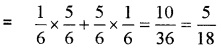P(X = 2) = P(six, six) = $$\frac{1}{6} \times \frac{1}{6}=\frac{1}{36}$$
The required Probability Distribution is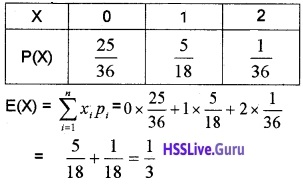Variance = σ2 = E(X2) – [E(X)]2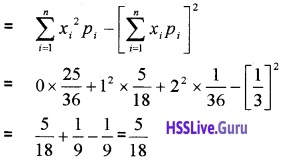Question 16.
A random variable X has the following probability distributionDetermine

1. k
2. P(X < 3)
3. P(X > 6)
4. P(0 < X < 3)

1. We know that sum of the probabilities is = 1
0 + k + 2k + 2k + 3k + k2 + 2k2 + 7k2 + k = 1
10k2 + 9k – 1 = 0
(k + 1)(10k – 1) = 0; k= -1 or k = $$\frac{1}{10}$$
(negative value cannot be accepted).

2. P(X < 3) = P(0) + P( 1) + P(2)
0 + k + 2k + 2k = 3k = $$\frac{3}{10}$$.

3. P(X < 3) = P(7) = 7k2 + k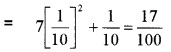4. P(0 < X < 3) = P(1) + P(2) = k + 2k = 3k = $$\frac{3}{10}$$. Question

Question 17.
(i) P(A) = $$\frac{7}{13}$$; P(B) = $$\frac{9}{13}$$; (A ∩ B) = $$\frac{4}{13}$$, then P(A/B) is
(a)  $$\frac{9}{4}$$
(b)  $$\frac{16}{13}$$
(c)  $$\frac{4}{9}$$
(d)  $$\frac{11}{13}$$
(ii) Probability of solving a specific problem independently by A and B are $$\frac{1}{2}$$ and $$\frac{1}{3}$$ respectively. If both try to solve the problem independently, then
(a) Find the probability that the problem is solved. (2)
(b) Find the probability that exactly one of them solve the problem. (1)
(i) (c) $$\frac{4}{9}$$

(ii)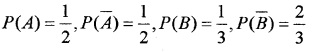P(Problem is solved)
= P(A ∪ B) = P(A) + P(B) – P(A ∩ B)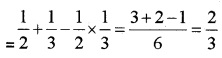(iii) P(Exactly one of them solve)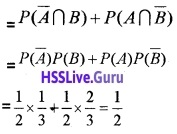### Plus Two Maths Probability Six Mark Questions and Answers Question

Question 1.
(i) A and B are two events such that P(A) = $$\frac{1}{5}$$ and P(A ∪ B) = $$\frac{2}{5}$$ Find P(B) if they are mutually exclusive
(a) $$\frac{1}{5}$$
(b) $$\frac{2}{5}$$
(c) $$\frac{3}{5}$$
(d) $$\frac{4}{5}$$
(ii) A box contains 3 red and 4 blue balls. Two balls are drawn one by one without replacement. Find the probability of getting both balls red.
(iii) Three cards are drawn successively without replacement from a pack of 52 cards. What is the probability that first two cards are queen and the third is king.
(i) (a) $$\frac{1}{5}$$.

(ii) Let A be the event that the first ball drawn is red and B be the event of drawing red ball in the second draw
P(A) = $$\frac{3}{7}$$
Probability of getting one red ball in the second draw = P(B/A) = $$\frac{2}{6}$$ = $$\frac{1}{3}$$. P(A ∩ B) = P(A).P(B/A)
$$=\frac{3}{7} \times \frac{1}{3}=\frac{1}{7}$$.

(iii) Let Q denote the event that the card drawn is Queen and K denote the event of drawing a King
P(Q) = $$\frac{4}{52}$$, P(Q/Q) = $$\frac{3}{51}$$
P(K/QQ) is the probability of drawing the third card is a king
P(K/QQ) = $$\frac{4}{50}$$
P(QQk) = P(Q)P(Q/Q)P(K/QQ)
$$=\frac{4}{52} \cdot \frac{3}{51} \cdot \frac{4}{50}=\frac{2}{5525}$$.Question 2.
60 shirts of different colours are on sale. If one shirt is chosen at random.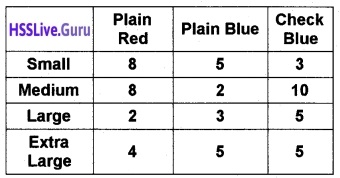1. What is the probability that it is red? (1)
2. What is the probability that it is plain and extra-large? (1)
3. What is the probability that it is small, given that it is blue? (2)
4. If A is the event ‘the shirt is medium’ and B is the event ‘the shirt is blue’. Are the events A and B independent? (2)

1. P(Red) = $$\frac{8+8+2+4}{60}=\frac{11}{30}$$.

2. P (Plain and extra-large) = $$\frac{4+5}{60}=\frac{3}{20}$$.

3. P(small/blue)4.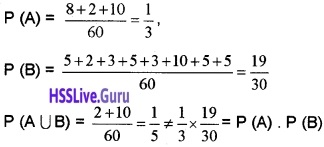∴ Not independent.

Question 3.
From a box containing balls numbered from, 1 to 100, one ball is drawn at random. The events X and Y are as follows. X: A perfect square is drawn. Y: An even number is drawn.

1. Find P(X) and P(Y). (2)
2. Compute P (X/Y). (2)
3. Are X and V independent? Justify. (2)

Perfect square numbered balls are
1, 4, 9, 16, 25, 36, 49, 64, 81, 100.
Therefore, there are 10 perfect square numbered balls, 50 even numbered balls and 5 perfect square even numbered balls.
1. P(X) = $$\frac{10}{100}=\frac{1}{10}$$, P(Y) = $$\frac{50}{100}=\frac{1}{2}$$
P (Even perfect square number) = P (X ∩ Y) $$\frac{50}{100}=\frac{1}{20}$$.

2. P(X/Y)
= P(Drawing a perfect square numbers from even numbers) = $$\frac{5}{50}=\frac{1}{10}$$.

3. We have, P (X ∩ Y) = $$\frac{1}{20}=\frac{1}{10} \times \frac{1}{2}$$
= P(X).P(Y).
Therefore X and Y are independent events.Question 4.
(i) The probability of three mutually exclusive events A, B, and C are given by 2/3, 1/4, 1/6 respectively. Is this statement ________ (1)
(a) true?
(b) false?
(c) cannot be said?
(d) data not sufficient?
A husband and wife appear in an interview for two vacancies in the same post. The probability of husband’s selection is 1/7 and that of wife’s selection is 1/5. What is the probability that
(ii) Only one of them will be selected? (3)
(iii) None will be selected? (2)
(i) (b) Probability should be less than or equal to 1.
Here A, B, C are mutually exclusive.
Then,
P(A ∪ B ∪ C) = P(A) + P(B) + P(C) = $$\frac{2}{3}+\frac{1}{4}+\frac{1}{6}=\frac{26}{24}>1$$
∴ statement is false.

(ii) H – Event of husband selected,
W – Event of wife selected(iii) P (None of them will be selected)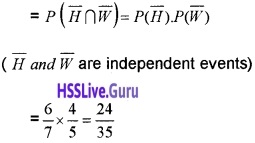Question 5.
(i) Find P(A∩B) if A and B are independent events with P(A) = $$\frac{1}{5}$$ and P(B) = $$\frac{5}{8}$$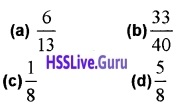(ii) An unbiased die is thrown twice. Let the event A be getting prime number in the first throw and B be the event of getting an even number in the second throw. Check the independence of the events A and B. (3)
(iii) The probability of solving a problem independently by A and B are $$\frac{1}{3}$$ and $$\frac{1}{4}$$ respectively. Find the probability that exactly one of them solves the problem. (2)
(i) (c) $$\frac{1}{8}$$.

(ii) P(A) = $$\frac{18}{36}$$ = $$\frac{1}{2}$$
P(B) = $$\frac{18}{36}$$ = $$\frac{1}{2}$$
P(A∩B) = P( prime number in first throw and even number in the second throw)∴ A and B are independent events.

(iii)Probability of exactly one of them solves the problem = P(A)P(B’) + P(B)P(A’)Question 6.
(i) A set of events E1 + E2,…….En are said to be a partition of the Sample Space, then which of the following conditions is always not true (1)
(a) E1∪ E2 ∪……..∪ En = S,
(b) E1 ∩ En = Φ,
(c) P(E1) > 0,
(d) P(E1) ≥ P(En)
(ii) A person has undertaken a business. The probabilities are 0.80 that there will be a crisis, 0.85 that the business will be completed on time if there is no crisis and 0.35 that the business will be completed on time if there is a crisis. Determine the probability that the business will be completed on time. (2)
(iii) A box contains 5 red and 10 black balls. A ball is drawn at random, its colour is noted and is returned to the box. More over 2 additional balls of the colour drawn are put in the box and then a ball is drawn. What is the probability that the second ball is red? (3)
(i) P(E1) ≥ P(En).

(ii) Let A be the event that the business will be completed on time and B be the event that there will be a crisis
P(B) = 0.80
P(no crisis) = P(B’) = 1 – P(B) = 0.20
P(A/B) = 0.35 P(A/B’) = 0.85
By theorem on total probability
P(A) = P(B)P(A/B) + P(B’)P(A/B’)
= 0.8 × 0.35 + 0.2 × 0.85 = 0.45.

(iii) Let a red ball be drawn in the first attempt P(drawing a red ball) = $$\frac{5}{15}=\frac{1}{3}$$
If two red balls are added to the box, then the box contains 7 red balls and 10 black balls
P(drawing a red ball) = $$\frac{7}{17}$$
Let a black ball be drawn in the first attempt P(drawing a black ball) = $$\frac{10}{15}=\frac{2}{3}$$
If two black balls are added to the box, then the box contains 5 red and 12 black balls
P(drawing a red ball) = $$\frac{5}{17}$$
Probability of drawing the second ball red isQuestion 7.

1. Bag I contains 5 red and 6 black balls. Bag II contains 7 red and 5 black balls. One ball is drawn at random from one of the bags and it is found to be red. Find the probability that it was drawn from bag I. (3)
2. A card from a pack of 52 cards is lost. From the remaining cards of the pack, two cards are drawn and are found to be both diamonds. Find the probability of the lost card being diamond. (3)

1. Let E1 be the event of choosing Bag I and E2 be the event of choosing Bag II
A be the event of drawing a red ball2. Let E1 be the event of choosing a diamond and E2 be the event of choosing a non diamond card
A be the event that a card is lostWhen a diamond card is lost, there are 12 diamond cards in 52 cards. Then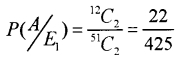When a non diamond card is lost, there are 13 diamond cards in 51 cards. Then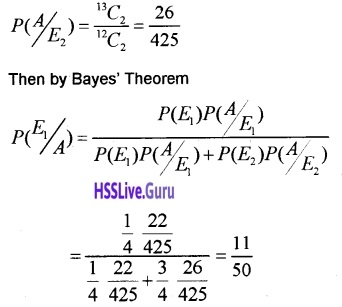Question 8.
(i) If X denotes number of heads obtained in tossing two coins. Then which of the following is false (1)
(a) X(HH) = 2
(b) X(HT) = 1
(c) X(TH)= 0
(d) X(TT) = 0
(ii) Find the probability distribution of the number of tails in the simultaneous toss of two coins. (2)
(iii) A coin is tossed so that the head is 3 times as likely to occur as tail. If the coin is tossed twice, find the probability distribution of number of tails. (3)
(i) (c) X(TH)= 0.

(ii) Sample space is S = {HH, HT, TH, TT}
Let X denote the number of tails, then
X(HH) = 2, X(HT) = 1, X(TH) = 1, X(TT) = 0
Therefore X can take the values 0, 1 or 2
P(HH) = P(HT) = P(TH) = P(TT) = $$\frac{1}{4}$$
P(X = 0) = $$\frac{1}{4}$$ P(X = 1) = $$\frac{1}{2}$$ P(X = 2) = $$\frac{1}{4}$$
Then the Probability distribution is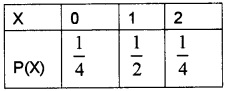(iii) Let the probability of getting a tail in the biased coin be x.
P(T) = x P(H) = 3x
P(T) + P(H) = 1 ⇒ x + 3x = 1 x = $$\frac{1}{4}$$
P(T) = $$\frac{1}{4}$$ P(H) = $$\frac{3}{4}$$
Let X denote the random variable representing the number of tails
P(X = 0) = P(HH) = P(H).P(H) = $$\frac{9}{16}$$
P(X = 1) = P(HT) + P(TH)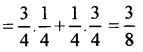P(X = 2) = P(TT) = P(T).P(T) = $$\frac{1}{16}$$
Then the Probability distribution is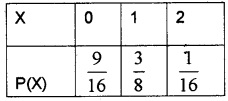Question 9.
If a fair coin is tossed 10 times, find the probability of

2. At least 6 heads. (2)
3. At most 6 heads. (2)

Let X denotes the random variable of number of heads in an experiment of 10 trials.
Clearly X has a Binomial Distribution with
n = 10 and p = $$\frac{1}{2}$$ ⇒ P(x) = nCxqn-x Px1. P(x = 6)2. P(at least 6 heads) = P(X ≥ 6)
= P(X = 6) + P(X = 1)+P(X = 8)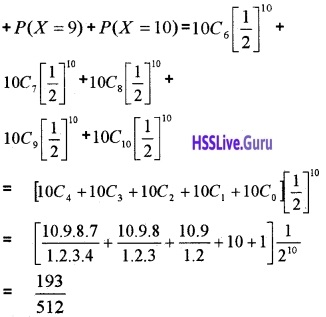P(at most 6 heads) = P(X ≤ 6)
= P(X = 6) + P(X = 5) + P(X = 4) + P(X = 3) + P(X = 2) + P(X = 1) + P(X = 0)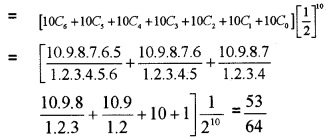Question 10.
Five cards are drawn successively with a replacement from a pack of 52 cards. What is the probability that

1. All the 5 cards are spades? (2)
2. only 3 cards are spade? (2)
3. none is a spade? (2)

Let X denotes the random variable of number of spades cards in an experiment of 5 trials. Clearly X has a Binomial Distribution with n = 5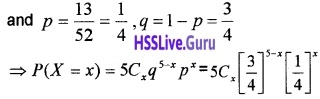1. P(all 5 are spades) = P(X = 5)2. P(3 are spades) = P(X = 3)3. P(non-spade) = P(X = 0) =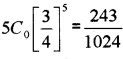Question 11.
Find the probability distribution, Mean and Variance of the number of success in two tosses of a die, where a success is defined as

1. number greater than 4. (3)
2. 6 appears on at least on die. (3)

1. Let X denotes the random variable of getting a 5, 6. Clearly X can take values 0, 1, 2.
When number 1, 2, 3, 4 appears in both die. Number of such cases = 4 × 4 = 16
P(X=0) = P(no success) = $$\frac{16}{36}=\frac{4}{9}$$
When 5, 6 in one die and other with 1, 2, 3, 4 and visa versa.
Number of such cases is 2 × 4 + 4 × 2 = 16.
P(X= 1) = P(1 success and 1 no success) = $$\frac{16}{36}=\frac{4}{9}$$
When number 5, 6 appears in Jjoth die. Number Hof such cases = 2 × 2 = 4.
P(success) = $$\frac{4}{36}=\frac{1}{9}$$
The required Probability Distribution is2. Let X denotes the random variable of getting at least 6 on one die. Clearly X can take values 0, 1.
No success means 1, 2, 3, 4, 5 appears on both die. Number of such cases is 5 × 5 = 25.
P(X=0) = P(no success) = $$\frac{25}{36}$$
When 6 in one die and other with 1, 2, 3, 4, 5 and visa versa. Number of such cases is 1 × 5 + 5 × 1 = 10
When both the die is 6. Number of such case is 1. Therefore total cases is 1 + 10 = 11
P(X=1) = P(1 success) = P(at least 1 six) = $$\frac{11}{36}$$
The required Probability Distribution isQuestion 12.
(i) If A and B are two events such that A ⊂ B and P(A) ≠ 0 then P(A/B) is (1)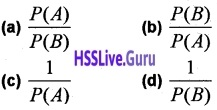(ii) There are two identical bags. Bag I contains 3 red and 4 black balls while Bag II contains 5 red and 4 black balls. One ball is drawn at random from one of the bags.
(a) Find the probability that all the ball drawn are red. (3)
(b) If the balls drawn is red what is the probability that it was drawn from Bag I? (2)
(i) Describe the events as follows.
A: ‘getting a red ball’.
E1: ‘choosing Bag I.’
E2: ‘choosing Bag II.’
P(E1) = P(E2) = $$\frac{1}{2}$$
P(A/E1) = P
(drawing a red ball from Bag I) = $$\frac{3}{7}$$
P(A/E2) = P
(drawing a red ball from Bag II) = $$\frac{5}{9}$$
P (All the balls drawn is red)
= P(A) = P(E1)P(A/E1) + P(E2)P(A/E2)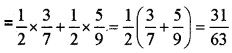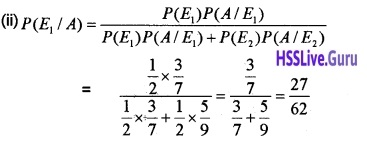Question 13.
Consider the following probability distribution of a random, variable X.1. Find the value of k. (2)
2. Determine the Mean and Variance of X. (4)